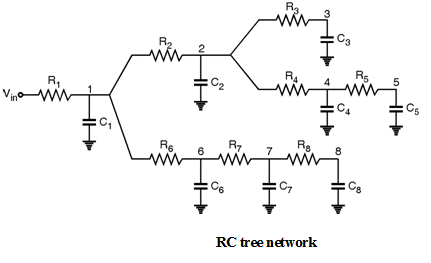Home > Digital CMOS Design > Propagation Delay > Elmore Delay Model

MOSFET-Fundamentals

Elmore Delay Model :

As we know that simple RC model provides general approximation of timing behavior of digital integrated circuits. In order to improve accuracy of RC model, Elmore delay model is used. Here, the RC segments made up of series resistance RN and a capacitance CNare created.

Consider RC network shown in Figure below connected in the form of RC segments.Now, here the Elmore delay at node 7 is calculated as,

```			D7	=	R1 C1 + R1 C2 + R1 C3 + R1 C4 + R1 C5 + (R1 + R6) C6
+ (R1 + R6 + R7) C7 + (R1 + R6 + R7) C8
```

In the same manner, the Elmore delay at node 5 is calculated as,

```	D5	=	R1 C1 + (R1 + R2) C2 + (R1 + R2) C3 + (R1 + R2 + R4) C4
+  (R1 + R2 + R4 + R5) C5 + R1 C6 + R1 C7 + R1 C8
```